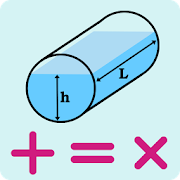# Tank Volume

### By Pixelsdo Mobile

Tank Volume Calculator is a fast and simple app for calculating volume of tanks. Also you can calculate liquid volume in a tank. İf you know density of liquid you can calculate weight of liquid too

Developed with an intuitive interface. It means less click, fast results. App remembers your settings for next use.

Tank volume calculator also has a built in volume convertor, if you need to convert volume types, like m3, liter, imp. Gallon, U.S Gallon or BBL

Calculations are performed for:

- Vertical tank

- Horizontal tank

- Rectangular tank

- Ecliptic tank

Other Features of Tank Volume Calculator

- Metric or imperial Units support

- Internet connection is not required.

- Small apk size.

- No background process.

- Share or Save function.

- Better tablet support.

- Fast and simple.

- Totally Free.

* This Calculator should be used as an estimating tool ONLY.

Application is not responsible for any discrepancies on calculations. *

Similar Apps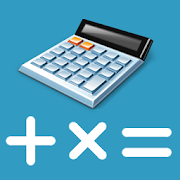##### Loan Calculator

Description:

Loan Calculator is a free mobile app that allows you to calculate all types of loan.SIMPLE, FAST AND INTUITIVE Many banks offer different conditions and you can not make your choice? Loan Calculator calculates the monthly payment, the amount of interest paid, the total cost of cr...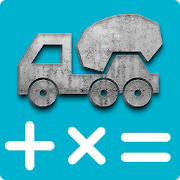##### Concrete Calculator

Description:

A simple tool to calculate how much concrete is needed for pouring slabs, footings, columns and steps.Concrete Calculator is a free calculator with the following functionalities:-Calculate cement, sand and aggregate amount in concrete.-How many premix concrete bags require...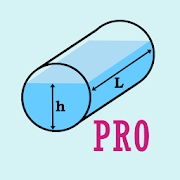##### Tank Volume Calculator Pro

Description:

Tank Volume Calculator is a fast and simple app for calculating volume of tanks. Also you can calculate liquid volume in a tank. İf you know density of liquid you can calculate weight of liquid tooDeveloped with an intuitive interface. It means less click, fast results. App remembers yo...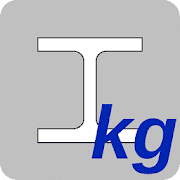##### Kouzaitap

Description:

【Introduction】This application calculates the weight(kg) and surface area(m2) of steel used in Japan (JIS Ferrous Materials Metallurgy).It also provides a calculation of cross-sectional shape and performance.【Functions】You can share, memorize, and e-mail calcula...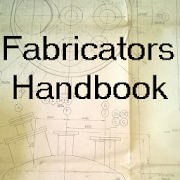##### Fabricators Handbook

Description:

Sheet metal pattern development tools for cones, segmental bends, set on pipes. Plus flange/ tube tables, various calculators and more.This could be the most useful tool in your toolbox. As a fabricator of 45 years, the last 30 or so as the workshop manager, I have consta...